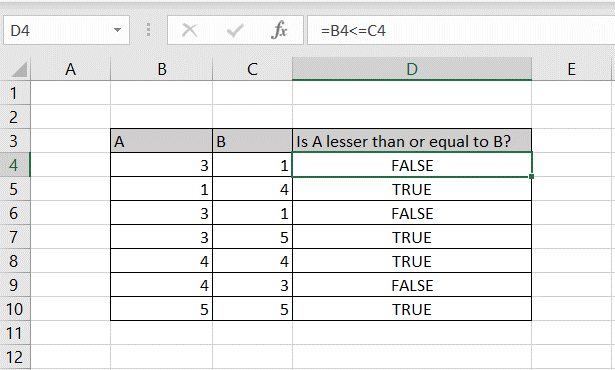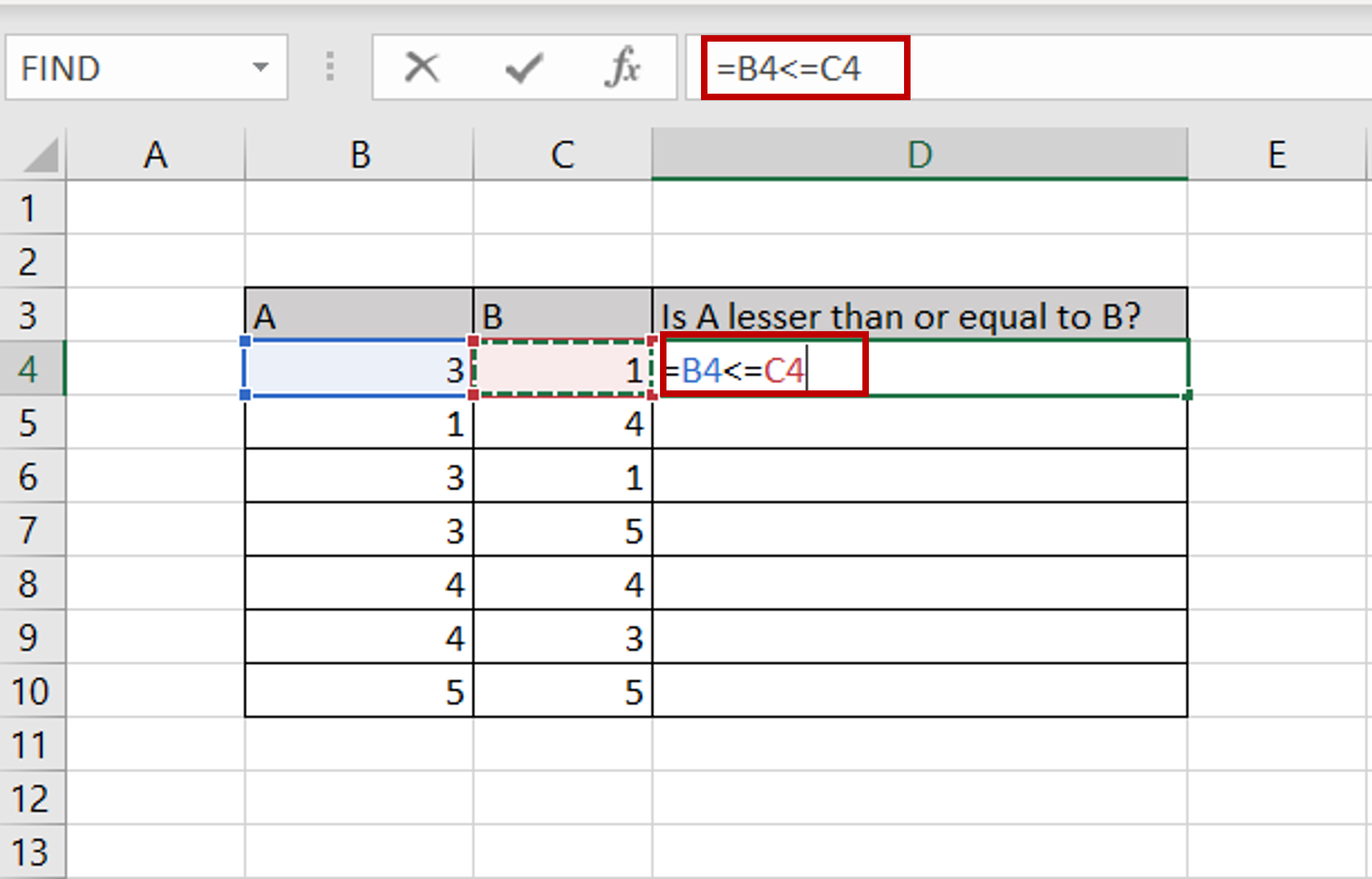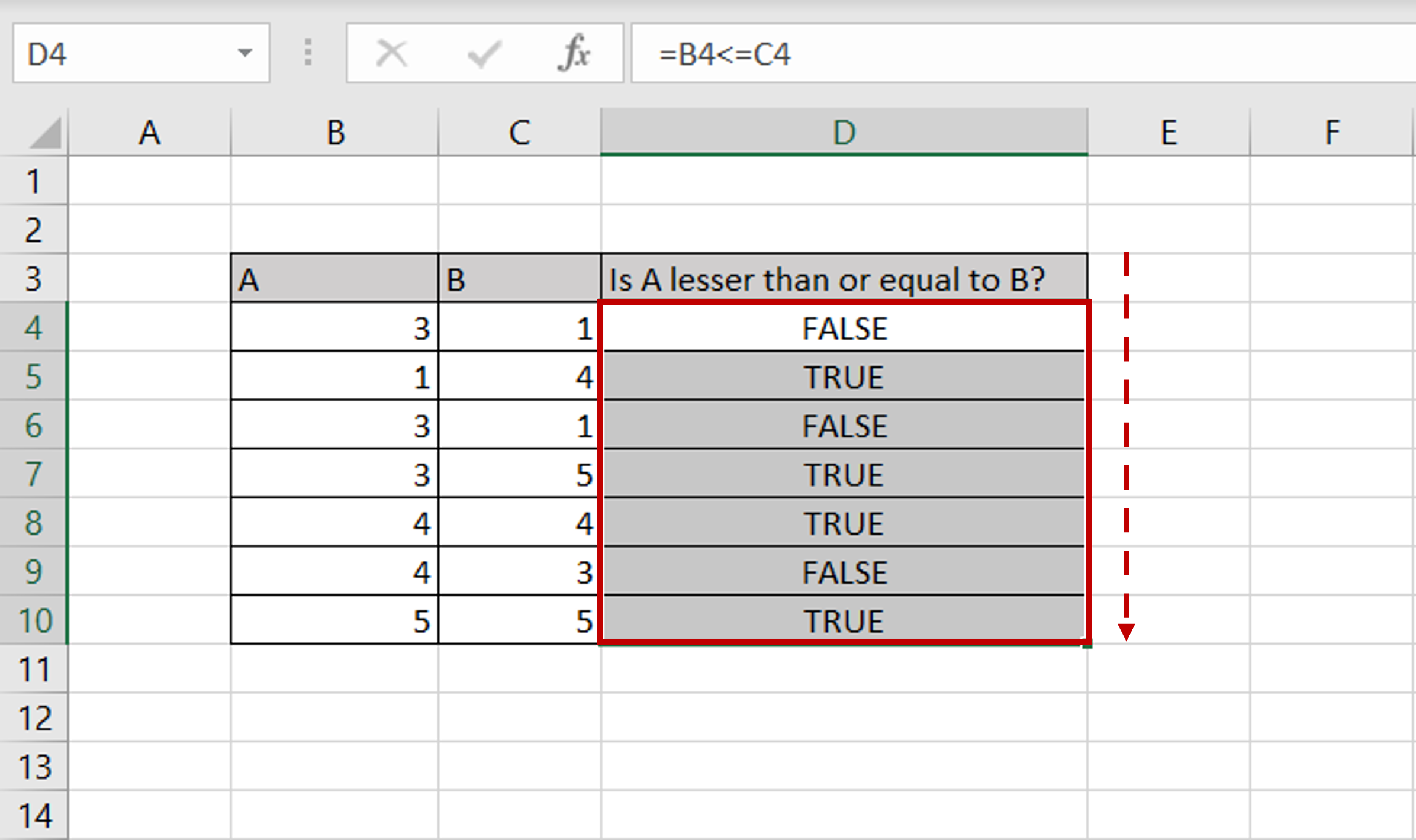# How to type lesser than or equal to in Excel

You can watch a video tutorial here.Excel is widely used for mathematical operations. You may have a formula in which you need to type the ‘lesser than or equal to’ symbol.

### Step 1 – Use ‘<’ with ‘=’– Use the ‘lesser than’ (>) symbol followed by the ‘equal sign’ (=) in the formula

### Step 2 – Copy the formula– Using the fill handle from the first cell, drag the formula to the remaining cells
OR
a) Select the cell with the formula and press Ctrl+C or choose Copy from the context menu (right-click)
b) Select the rest of the cells in the column and press Ctrl+V or choose Paste from the context menu (right-click)